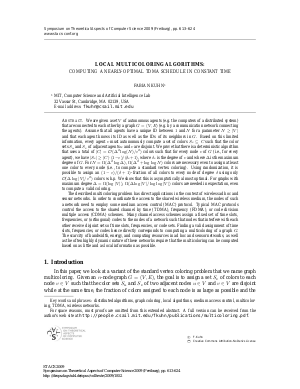Document# Local Multicoloring Algorithms: Computing a Nearly-Optimal TDMA Schedule in Constant Time

### Author Fabian Kuhn## File

LIPIcs.STACS.2009.1852.pdf
• Filesize: 144 kB
• 12 pages

## Cite As

Fabian Kuhn. Local Multicoloring Algorithms: Computing a Nearly-Optimal TDMA Schedule in Constant Time. In 26th International Symposium on Theoretical Aspects of Computer Science. Leibniz International Proceedings in Informatics (LIPIcs), Volume 3, pp. 613-624, Schloss Dagstuhl - Leibniz-Zentrum für Informatik (2009)
https://doi.org/10.4230/LIPIcs.STACS.2009.1852

## Abstract

We are given a set $V$ of autonomous agents (e.g.\ the computers of a distributed system) that are connected to each other by a graph $G=(V,E)$ (e.g.\ by a communication network connecting the agents). Assume that all agents have a unique ID between $1$ and $N$ for a parameter $N\ge|V|$ and that each agent knows its ID as well as the IDs of its neighbors in $G$. Based on this limited information, every agent $v$ must autonomously compute a set of colors $S_v\subseteq C$ such that the color sets $S_u$ and $S_v$ of adjacent agents $u$ and $v$ are disjoint. We prove that there is a deterministic algorithm that uses a total of $|C|=\ensuremath{\mathcal{O}}(\Delta^2\log(N)/\ensuremath{\varepsilon}^2)$ colors such that for every node $v$ of $G$ (i.e., for every agent), we have $|S_v|\ge |C|\cdot(1-\ensuremath{\varepsilon})/(\delta_v+1)$, where $\delta_v$ is the degree of $v$ and where $\Delta$ is the maximum degree of $G$. For $N=\Omega(\Delta^2\log\Delta)$, $\Omega(\Delta^2+\log\log N)$ colors are necessary even to assign at least one color to every node (i.e., to compute a standard vertex coloring). Using randomization, it is possible to assign an $(1-\ensuremath{\varepsilon})/(\delta+1)$-fraction of all colors to every node of degree $\delta$ using only $\ensuremath{\mathcal{O}}(\Delta\log|V|/\ensuremath{\varepsilon}^2)$ colors w.h.p. We show that this is asymptotically almost optimal. For graphs with maximum degree $\Delta=\Omega(\log|V|)$, $\Omega(\Delta\log|V|/\log\log|V|)$ colors are needed in expectation, even to compute a valid coloring. The described multicoloring problem has direct applications in the context of wireless ad hoc and sensor networks. In order to coordinate the access to the shared wireless medium, the nodes of such a network need to employ some medium access control (MAC) protocol. Typical MAC protocols control the access to the shared channel by time (TDMA), frequency (FDMA), or code division multiple access (CDMA) schemes. Many channel access schemes assign a fixed set of time slots, frequencies, or (orthogonal) codes to the nodes of a network such that nodes that interfere with each other receive disjoint sets of time slots, frequencies, or code sets. Finding a valid assignment of time slots, frequencies, or codes hence directly corresponds to computing a multicoloring of a graph $G$. The scarcity of bandwidth, energy, and computing resources in ad hoc and sensor networks, as well as the often highly dynamic nature of these networks require that the multicoloring can be computed based on as little and as local information as possible.
##### Keywords
• Distributed algorithms
• Graph coloring
• Local algorithms
• Medium access control
• Multicoloring
• TDMA
• Wireless networks

## Metrics

• Access Statistics
• Total Accesses (updated on a weekly basis)
0